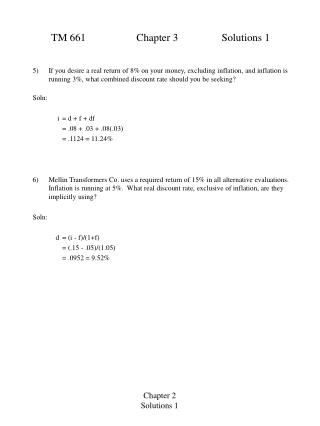DownloadDownload PresentationTM 661 Chapter 3 Solutions 1

TM 661 Chapter 3 Solutions 1

Télécharger la présentationTM 661 Chapter 3 Solutions 1

- - - - - - - - - - - - - - - - - - - - - - - - - - - E N D - - - - - - - - - - - - - - - - - - - - - - - - - - -
Presentation Transcript

1. TM 661 Chapter 3 Solutions 1 5) If you desire a real return of 8% on your money, excluding inflation, and inflation is running 3%, what combined discount rate should you be seeking? Soln: i = d + f + df = .08 + .03 + .08(.03) = .1124 = 11.24% 6) Mellin Transformers Co. uses a required return of 15% in all alternative evaluations. Inflation is running at 5%. What real discount rate, exclusive of inflation, are they implicitly using? Soln: d = (i - f)/(1+f) = (.15 - .05)/(1.05) = .0952 = 9.52% Chapter 2 Solutions 1

2. TM 661 Chapter 3 Solutions 1 8) A landfill has a first cost of \$270,000. Annual operating and maintenance costs for the first year will be \$40,000. These costs will increase at 11% per year. Income for dumping rights at the landfill will be held fixed at \$120,000 per year. The landfill will be in operation for 10 years. Inflation will average 8%, and a real return of 3.6% is desireed. A) determine the present worth using then-current \$. B) determine the present worth using contant worth \$ Soln: Then current \$ are the actual physical cashflow in periods 0-10. The net present value is then computed at the combined interest rate i = d + f + df = 11.89%. Constant worth \$ are computed by taking the physical cash flow (then current) and discounting them back to time 0 by the inflation rate. The net present value is then computed by using the real interest rate d = 3.6%. The cash flow streams and net present value are shown below. Chapter 2 Solutions 2

3. 2,500 125 125 125 1 2 3 16 P TM 661 Chapter 3 Solutions 1 16) Dr. Schulz is considering purchasing a bond having a face value of \$2,500 and a bond rate of 10% payable semi-annually. The bond has a remaining life of 8 years. How much should she pay for the bond in order to earn a return on investment of 14% compounded semiannually? Soln: The bond earns 10% per year or 5% semi-annually. 5% of 2,500 = 125. 8 years remaining equates to 16 semi-annual periods. The physical cash flow for the bond is then shown below. Since Dr. Schulz wants 14% compounded semi-annually (7%) per period, we can compute the Present worth of the cash flow by = 125 (P/A, 7, 6) + 2,500 (P/F, 7, 16) = 125 (9.4466) + 2,500 (.3387) = 2,027 Chapter 2 Solutions 3